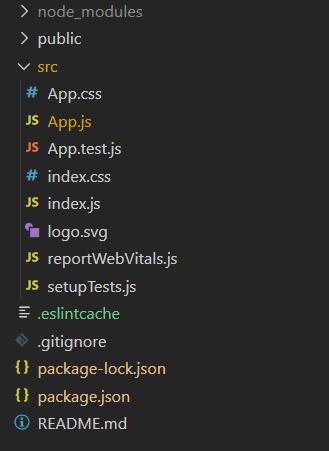GFG App
Open AppBrowser
Continue

# ReactJS useCallback Hook

The useCallback hook is used when you have a component in which the child is rerendering again and again without need.

Pass an inline callback and an array of dependencies. useCallback will return a memoized version of the callback that only changes if one of the dependencies has changed. This is useful when passing callbacks to optimized child components that rely on reference equality to prevent unnecessary renders.

Syntax:

```const memoizedCallback = useCallback(
() => {
doSomething(a, b);
},
[a, b],
);```

Creating React Application:

Step 1: Create a React application using the following command.

`npx create-react-app foldername`

Step 2: After creating your project folder i.e. foldername, move to it using the following command.

`cd foldername`

Project Structure: It will look like the following.Without useCallback Hook: The problem is that once the counter is updated, all three functions are recreated again. The alert increases by three at a time but if we update some states all the functions related to that states should only re-instantiated. If another state value is unchanged, it should not be touched. Here, the filename is App.js

## Javascript

 `import React, { useState, useCallback } from ``'react'` `const funccount = ``new` `Set();` `const App = () => {`     `  ``const [count, setCount] = useState(0)` `  ``const [number, setNumber] = useState(0)`   `  ``const incrementCounter = () => {` `    ``setCount(count + 1)` `  ``}` `  ``const decrementCounter = () => {` `    ``setCount(count - 1)` `  ``}` `  `  `   ``const incrementNumber = () => {` `    ``setNumber(number + 1)` `  ``}` `  `  `funccount.add(incrementCounter);` `funccount.add(decrementCounter);` `funccount.add(incrementNumber);` `alert(funccount.size);`   `  ``return` `(` `    ``
` `      ``Count: {count}` `      ``` `      ``` `      ``` `    ``
` `  ``)` `}`     `export ``default` `App;`

With useCallback hook: To solve this problem we can use the useCallback hook. Here, the filename is App.js.

## Javascript

 `import React, { useState, useCallback } from ``'react'` `var` `funccount = ``new` `Set();` `const App = () => {`     `  ``const [count, setCount] = useState(0)` `  ``const [number, setNumber] = useState(0)`   `const incrementCounter = useCallback(() => {` `  ``setCount(count + 1)` `}, [count])` `const decrementCounter = useCallback(() => {` `  ``setCount(count - 1)` `}, [count])` `const incrementNumber = useCallback(() => {` `  ``setNumber(number + 1)` `}, [number])` `  `  `funccount.add(incrementCounter);` `funccount.add(decrementCounter);` `funccount.add(incrementNumber);` `alert(funccount.size);`   `  ``return` `(` `    ``
` `      ``Count: {count}` `      ``` `      ``` `      ``` `    ``
` `  ``)` `}`     `export ``default` `App;`

Output: As we can see from the below output when we change the state ‘count’ then two functions will re-instantiated so the set size will increase by 2 and when we update the state ‘number’ then only one function will re-instantiated and the size of the set will increase by only one.My Personal Notes arrow_drop_up